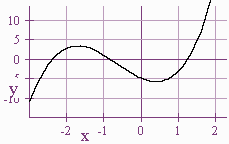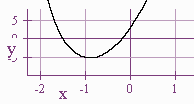$$\newcommand{\id}{\mathrm{id}}$$ $$\newcommand{\Span}{\mathrm{span}}$$ $$\newcommand{\kernel}{\mathrm{null}\,}$$ $$\newcommand{\range}{\mathrm{range}\,}$$ $$\newcommand{\RealPart}{\mathrm{Re}}$$ $$\newcommand{\ImaginaryPart}{\mathrm{Im}}$$ $$\newcommand{\Argument}{\mathrm{Arg}}$$ $$\newcommand{\norm}{\| #1 \|}$$ $$\newcommand{\inner}{\langle #1, #2 \rangle}$$ $$\newcommand{\Span}{\mathrm{span}}$$

3.3: Polynomial Equations

$$\newcommand{\vecs}{\overset { \rightharpoonup} {\mathbf{#1}} }$$

$$\newcommand{\vecd}{\overset{-\!-\!\rightharpoonup}{\vphantom{a}\smash {#1}}}$$

So far we have learned how to find the roots of a polynomial equation. If we have an equation that involves only polynomials we follow the steps:

1. Bring all the terms over to the left hand side of the equation so that the right hand side of the equation is a 0.
2. Get rid of denominators by multiplying by the least common denominator.
3. If there is a common factor for all the terms, factor immediately. Otherwise, multiply the terms out.
4. Use a calculator to locate roots.
5. Use the Rational Root Theorem and synthetic division to exactly determine the roots.

Example 1

Find all the rational solutions of

$\dfrac{(2x^3 - 5)}{4} = x - x^2.$Solution:

1. $$\dfrac{(2x^3 - 5)}{4} - x + x^2 = 0$$

2. $$(2x^3 - 5)- 4x + 4x^2 = 0$$

3. $$2x^3 + 4x^2 - 4x - 5 = 0$$

4. From the graph, we see that there is a root between -3 and -2 and a root between 0 and -1 and a root between 1 and 2.

5. Since the only possible rational roots are 1, -1, 5, -5, .5, -.5, 2.5, -2.5, the possible rational roots are $$-\dfrac{5}{2}$$ and -.5. Neither of these two are roots, hence there are no rational roots.

Example 2

Solve

$x[x^2(2x + 3) + 10x + 17] + 5 = 2.$1. $$x[x^2(2x + 3) + 10x + 17] + 3 = 0$$

2. $$2x^4 + 3x^3 + 10x^2 + 17x + 3 = 0$$

3. We see that there is a root between -2 and -1 and between -1 and 0.

4. Our only possible roots are $$-\frac{1}{2}$$ and $$-\frac{3}{2}$$.

5. Using synthetic division, we see that $$-\frac{3}{2}$$ is a root, and the remainder is

$2x^3 + 10x + 2 = 2(x^3 + 5x + 1)$

which has no rational roots. Hence the rational root is $$-\frac{3}{2}$$ and using the calculator we see that the irrational root is 0.198.

Larry Green (Lake Tahoe Community College)

• Integrated by Justin Marshall.